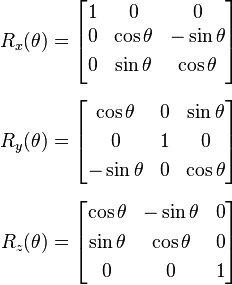# Rotational Matrix

Hi
I’m not very good at maths. There are too many gaps in my understanding of things like trigonometry etc.

I need a cheat sheet for some rotational matrixes for localPosition method in bge.

How do I rotate 90 degrees on the z axis?
How do I rotate -90 degrees on the z axis?

Thanks

I’m unsure of what exactly you’re asking for. If you want to purely rotate an object by 90 degrees on the Z axis (globally), then you can just use the object’s applyRotation() function. Use math.radians() to convert 90 degrees into radians, or use math.pi to do it yourself (since pi is 180 degrees, half of pi is 90 degrees).

``````

from bge import logic

import math

cont = logic.getCurrentController()

owner = cont.owner

owner.applyRotation([0, 0, math.pi / 2])

``````

Hi SolarLune, I solved my problem avoiding rotation altogether. Thanks though, that will likely be usefull for me in the future.

I meant the parameters for the localOrientation method not the localPosition method mentioned incorrectly in my original post.

obj.localOrientation = ([1.0, 0.0, 0.0], [0.0, 1.0, 0.0], [0.0, 0.0, 1.0])
I’m just clueless as to calculating matrix’s to set the orientation.

If you are desperate to use the matrix:

``````import bge, math
from bge import logic
from math import cos, sin

cont = logic.getCurrentController()
own = cont.owner

a = own['a']

def z():
own.worldOrientation = cos(a)
own.worldOrientation = -sin(a)
own.worldOrientation = 0

own.worldOrientation = sin(a)
own.worldOrientation = cos(a)
own.worldOrientation = 0

own.worldOrientation = 0
own.worldOrientation = 0
own.worldOrientation = 1

own['a'] += 0.5

z()

``````

a is the angle you want to change it by.
Here is it in a blend file all set up.
zMatrix.blend (478 KB)That is straight from Wikipedia,here’s the link.

BUT don’t do it this way. It’s ridiculously overkill when you could do it the way Solarlune suggested.

### Attachments

zMatrix.blend (489 KB)

I explain the basics of the orientation matrix in this video: https://www.youtube.com/watch?v=hyxS4RyWxpk#t=2m32s

With the above explanations you now know that rotation around the Z-Axis changes the X and Y components of the Matrix (which is not complete btw. but sufficient for rotation).

As you want to rotate in 90° steps the calculation of sin and cos is pretty simple (school math).

sin(90°) = 1
cos(90°) = 0

it means this are special cases making life a bit easier ;).

now fill it in the above rotation matrix around Z:

``````
0 -1  0
1  0  0
0  0  1

``````

to rotate -90°:

``````
0  1  0
-1  0  0
0  0  1

``````

Now you multiply this “90° around Z” rotation matrix with the existing orientation matrix to get the rotated orientation.